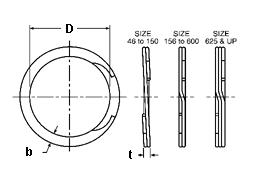### ZST-170

Display Units: inches |  metric
Request Quick Quote for this part.
 Ring Specs (D) Free Diameter: 6.363 +0.000 / -0.060 in. (t) Thickness: 0.165 +0.005 / -0.005 in. (b) Radial Wall: 0.312 +0.004 / -0.008 in. Groove Specs: (B) Application Diameter: 6.693 in. (G) Groove Diameter: 6.409 +0.008 / -0.008 in. (W) Groove Width: 0.174 +0.008 / -0.000 in. Groove Depth: 0.142 in. Other Specs Approximate Weight per 1000: (m) Material Thickness: 0.054 in. (N) Ring Number of Turns: 3.000 (Pg) Theoretical Thrust Load Capacity - Groove Yield:Notes: Yield Strength of Groove Material (Ys): 45,000 psi. Calculated using a safety factor (K) of 2Equation:Pg = [ B * d * Ys * pi ] / K    = [ (6.693 in.) * (0.142 in.) * (45,000 psi) * 3.14 ] / 2 67,146 lbs. (Pr) Theoretical Thrust Load Capacity - Ring Shear:Notes: Ring Material: Carbon Spring Steel (SAE 1070-1090). Shear Strength of Ring Material (Ss): 120,000 psi. Calculated using a safety factor (K) of 3Equation:Pr = [ B * t * Ss * pi ] / K    = [ (6.693 in.) * (0.165 in.) * (120,000 psi) * 3.14 ] / 3 138,705 lbs. Industry Equivalent Part Number(s):ZST-170